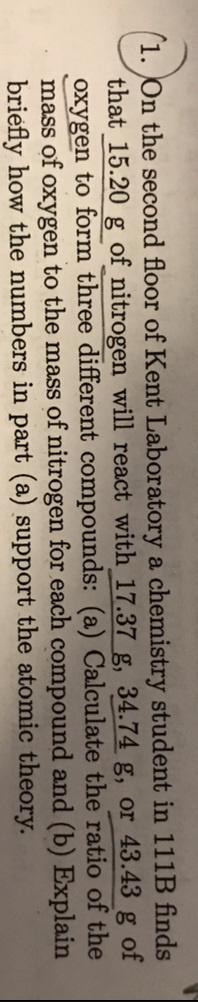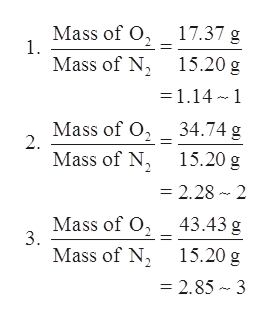# (1. On the second floor of Kent Laboratory a chemistry student in 111B findsthat 15.20 g of nitrogen will react with 17.37 g, 34.74 g, or 43.43 g ofoxygen to form three different compounds: (a) Calculate the ratio of themass of oxygen to the mass of nitrogen for each compound and (b) Explainbriefly how the numbers in part (a) support the atomic theory.

Question
68 viewshelp_outlineImage Transcriptionclose(1. On the second floor of Kent Laboratory a chemistry student in 111B finds that 15.20 g of nitrogen will react with 17.37 g, 34.74 g, or 43.43 g of oxygen to form three different compounds: (a) Calculate the ratio of the mass of oxygen to the mass of nitrogen for each compound and (b) Explain briefly how the numbers in part (a) support the atomic theory. fullscreen
check_circle

Step 1

Given information:

Mass of nitrogen = 15.20 g

Mass of oxygen for different compounds = 17.37 g, 34.74 g, and 43.43 g

Step 2

a. The ratio of mass of oxygen to the mass of nitrogen for each compound will be calculated as follows:help_outlineImage Transcriptionclose17.37 g Mass of O 1. 15.20 g Mass of N =1.14 1 34.74 g Mass of O 2 Mass of N 15.20 g = 2.28 2 Mass of O 3. 43.43 g 15.20 g Mass of N = 2.85 3 fullscreen
Step 3

b. Postulates for atomic theory are given as follows:

1. Matter is made up of indivisible particles called atoms.
2. All atoms of an element have identical mass and chemical properties.
3. Atoms of different elements have different mass and chemical properties.
4. Atoms combi...

### Want to see the full answer?

See Solution

#### Want to see this answer and more?

Solutions are written by subject experts who are available 24/7. Questions are typically answered within 1 hour.*

See Solution
*Response times may vary by subject and question.
Tagged in

### General Chemistry